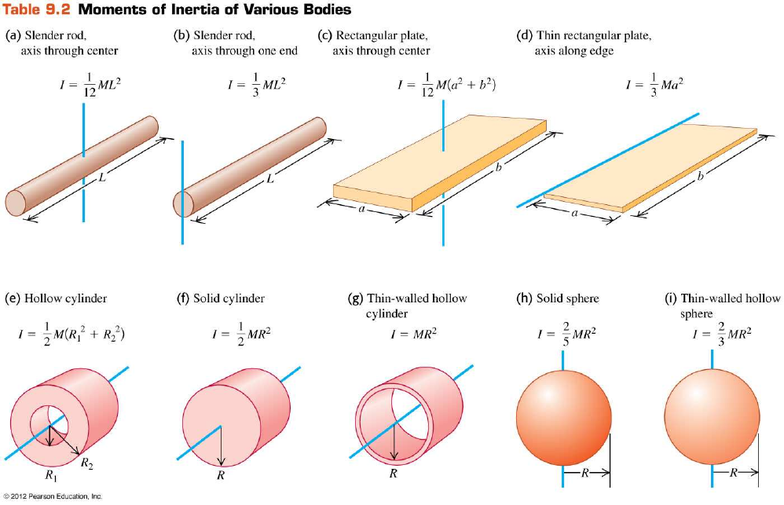# PHYSICS 140 Study Guide - Final Guide: Notecards, Scantron Corporation, Plane Wave

55 views16 pages
School
U of M
Department
Physics
Course
PHYSICS 140
Professor
Physics 140, Fall 2016
Final Exam
December 16, 2016
Form 1
Last First
Fill in your name, student ID number, and form number on the scantron and in the spaces above.
All cell phones, text messaging devices, computers, and communication devices of any type must be
turned off and stowed out of sight.
The exam is closed book. You may use four 3" × 5" (7.5 cm × 12.5 cm) notecards (both sides). You
may also use a calculator that cannot communicate with the outside world.
You cannot share calculators, notecards, scratch paper, exam booklets, or scantrons. Exam responses
must be based on your individual work only.
You must mark the correct answer on the scantron to get credit for each problem.
The exam is 120 minutes long. There are 25 multiple-choice questions. All questions are of equal
value. There is no penalty for guessing.
At the end of the exam, hand in your scantron and show your UM ID.
Constants and equations you may find useful:
x = x
0
+ v
0x
t + a
x
t
2
/2
v
x
= v
0x
+ a
x
t
v
x2
= v
0x2
+ 2a
x
(xx
0
)
θ = θ
0
+ ω
0z
t + α
z
t
2
/2
ω
z
= ω
0z
+ α
z
t
ω
z2
= ω
0z2
+ 2α
z
(θθ
0
)
v = Rω
a
tan
= dv/dt = Rα
a
= v
2
/R = ω
2
R
v
A/C
= v
A/B
+ v
B/C
(continued on the next page)
Unlock document

This preview shows pages 1-3 of the document.
Unlock all 16 pages and 3 million more documents.(continued from the previous page)
I = m
1
r
12
+ m
2
r
22
+ ··· = r
2
dm
I = I
CM
+ Md
2
F
k
=
k
N
F
s,x
= – kx
F
g
= Gm
1
m
2
/r
2
v
orb
= (GM/r)
1/2
v
esc
= (2GM/r)
1/2
 =
·
p = mv
=∆ =
 =

∆
r
CM
= (m
1
r
1
+ m
2
r
2
+ ···)/(m
1
+ m
2
+ ···)
τ = r × F
L = r × p = Iω
F
x
= – dPE/dx
TKE = mv
2
/2
RKE = Iω
2
/2
GPE = – Gm
1
m
2
/r
EPE = kx
2
/2
x(t) = Acos(ωt + φ)
a
x
= – ω
2
x
ω = (k/m)
1/2
or (g/L)
1/2
or (mgd/I
pivot
)
1/2
ω = 2πf = 2π/T
k = 2π/λ
v = ω/k = λ/T = λf = (F/)
1/2
P
aver
= ½(F)
1/2
ω
2
A
2
= ½vω
2
A
2
p
d
= p
0
+ ρgd
F
b
= ρ
fluid
V
displaced
g
A
1
v
1
= A
2
v
2
p
1
+ ρgy
1
+ ½ρv
12
= p
2
+ ρgy
2
+ ½ρv
22
g = 9.8 m/s
2
G = 6.67 × 10
-11
m
2
/kg
2
R
Earth
= 6.38 × 10
6
m
M
Earth
= 5.97 × 10
24
kg
p
atm
= 1 atm = 1.013 × 10
5
Pa
ρ
pure_water
= 1000 kg/m
3
TKE
i
+ RKE
i
+ GPE
i
+ EPE
i
+ W
non-cons
= TKE
f
+ RKE
f
+ GPE
f
+ EPE
f
Unlock document

This preview shows pages 1-3 of the document.
Unlock all 16 pages and 3 million more documents.

1. Which equation describes a plane wave travelling in the direction along the negative x axis?
a) cos+
b) cos+
c) coscos
d) cos
e) cos+
2. A string of mass 2.4 × 10
–3
kg and length 0.60 m vibrates transversely with a fundamental frequency
of 100 Hz. What is the tension in this string?
a) 0.16 N
b) 0.32 N
c) 13 N
d) 26 N
e) 58 N
Unlock document

This preview shows pages 1-3 of the document.
Unlock all 16 pages and 3 million more documents.

## Document Summary

Fill in your name, student id number, and form number on the scantron and in the spaces above. All cell phones, text messaging devices, computers, and communication devices of any type must be turned off and stowed out of sight. You may use four 3" 5" (7. 5 cm 12. 5 cm) notecards (both sides). You may also use a calculator that cannot communicate with the outside world. You cannot share calculators, notecards, scratch paper, exam booklets, or scantrons. Exam responses must be based on your individual work only. You must mark the correct answer on the scantron to get credit for each problem. At the end of the exam, hand in your scantron and show your um id. 2 = v0x x = x0 + v0xt + axt2/2 vx = v0x + axt. Z v = r atan = dv/dt = r arad = v2/r = 2r va/c = va/b + vb/c.